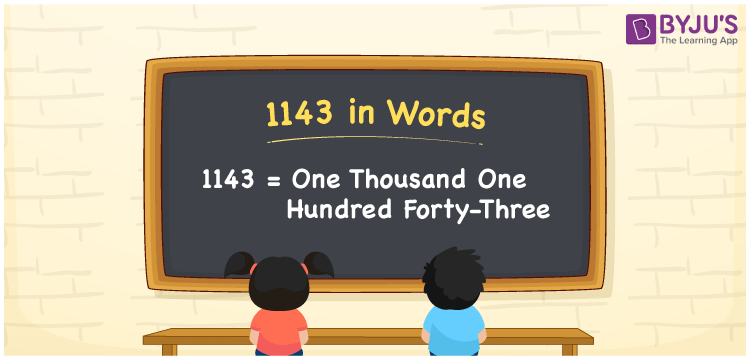# 1143 in Words

1143 in words is written as One thousand one hundred forty-three. In both the International System of Numerals and the Indian System of Numerals, 1143 is written as One thousand one hundred forty-three. The number 1143 is a Cardinal Number as it represents some quantity. For example, “the dress costs 1143 rupees.”

 1143 in Words One thousand one hundred forty-three One thousand one hundred forty-three in Number 1143

## 1143 in English Words

1143 in English words is read as “One thousand one hundred forty-three”.## How to Write 1143 in Words?

To write 1143 in words, we shall use the place value chart. In the place value chart, put 1 in the thousands, 1 in the hundreds, 4 in the tens and 3 in the ones, respectively. Let us make a place value chart to write the number 1143 in words.

 Thousands Hundreds Tens Ones 1 1 4 3

Thus, we can write the expanded form as

1 × Thousand + 1 × Hundred + 4 × Ten + 3 × One

= 1 × 1000 + 1 × 100 + 4 × 10 + 3 × 1

= 1000 + 100 + 40 + 3

= 1143

= One thousand one hundred forty-three.

1143 is a natural number, the successor of 1142 and the predecessor of 1144.

1143 in words – One thousand one hundred forty-three

• Is 1143 an odd number? – Yes
• Is 1143 an even number? – No
• Is 1143 a perfect square number? – No
• Is 1143 a perfect cube number? – No
• Is 1143 a prime number? – No
• Is 1143 a composite number? – Yes

## Frequently Asked Questions on 1143 in Words

Q1

### How to write 1143 in words?

1143 in words is written as One thousand one hundred forty-three.
Q2

### How to write 1143 in words in the International and Indian System of Numerals?

In both, the system of numerals, 1143 in words, is written as One thousand one hundred forty-three.
Q3

### How to write 1143 in a place value chart?

In the place value chart, write 1 in the thousands, 1 in the hundreds, 4 in the tens and 3 in the ones, respectively.